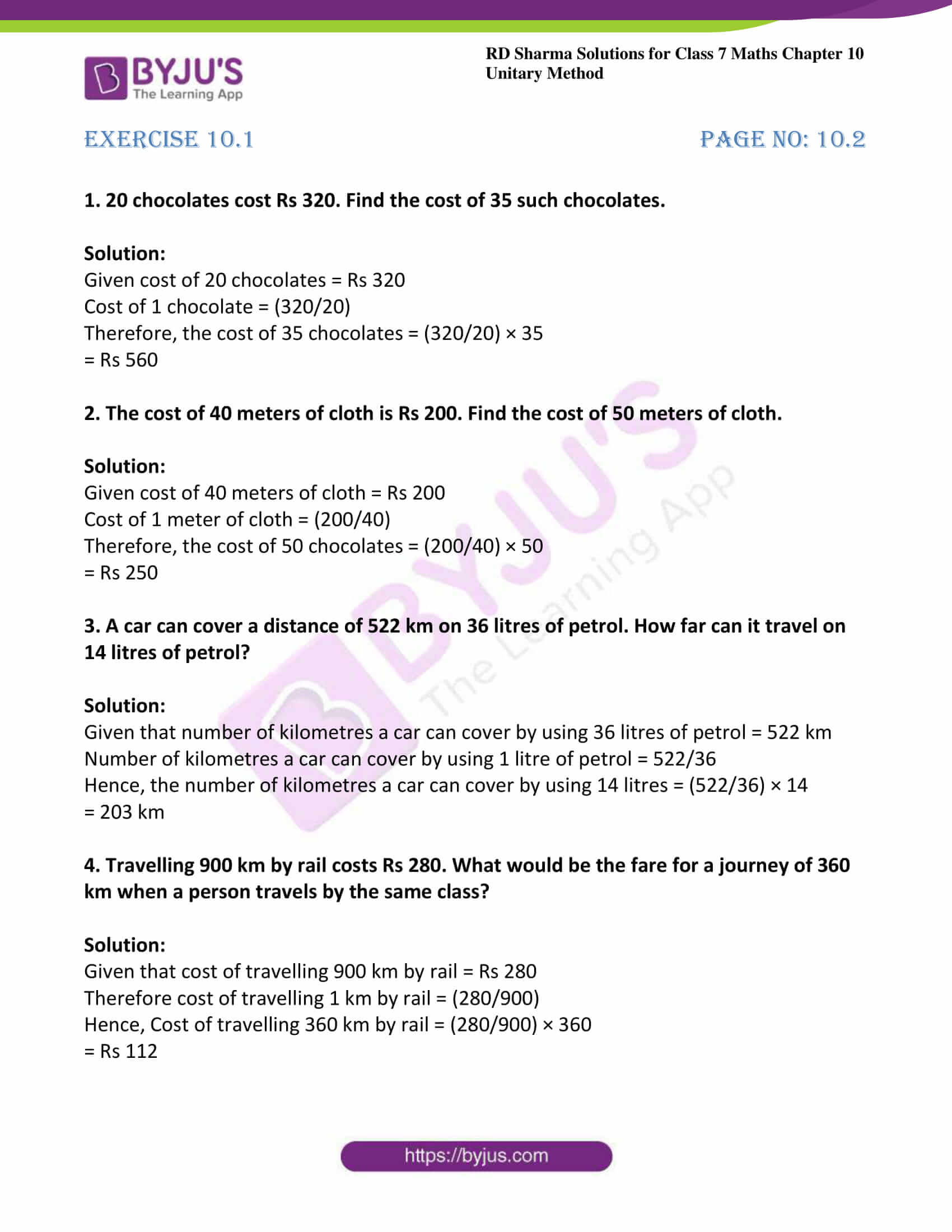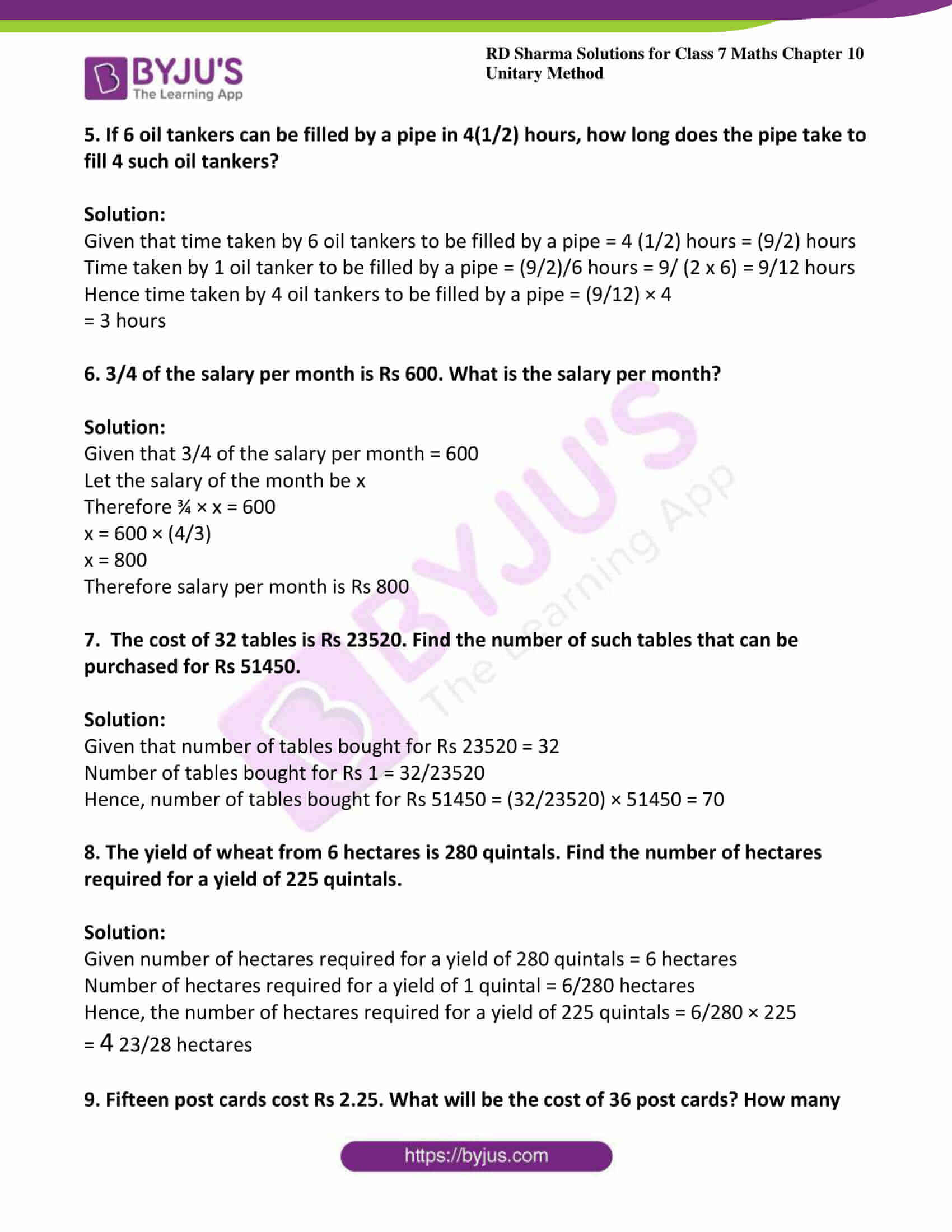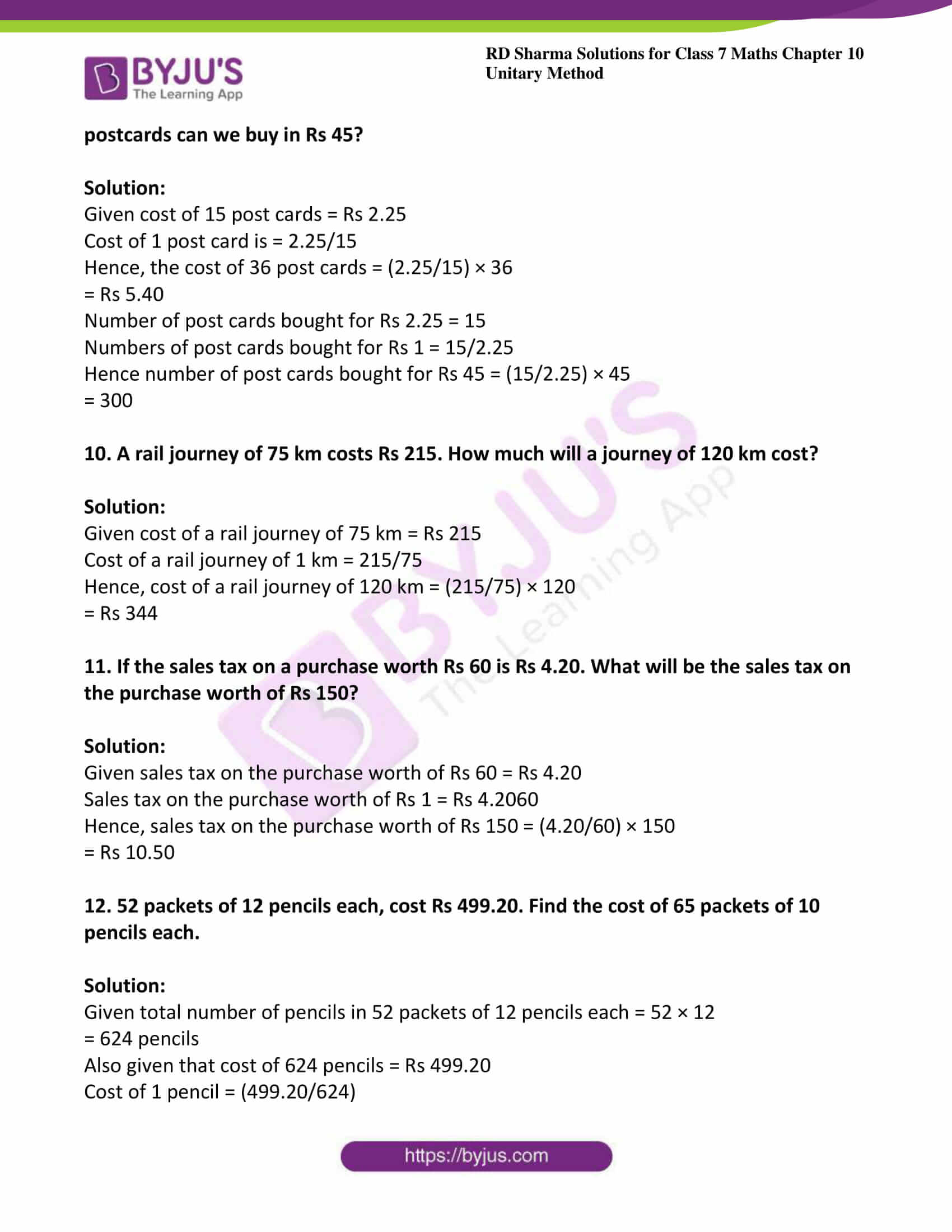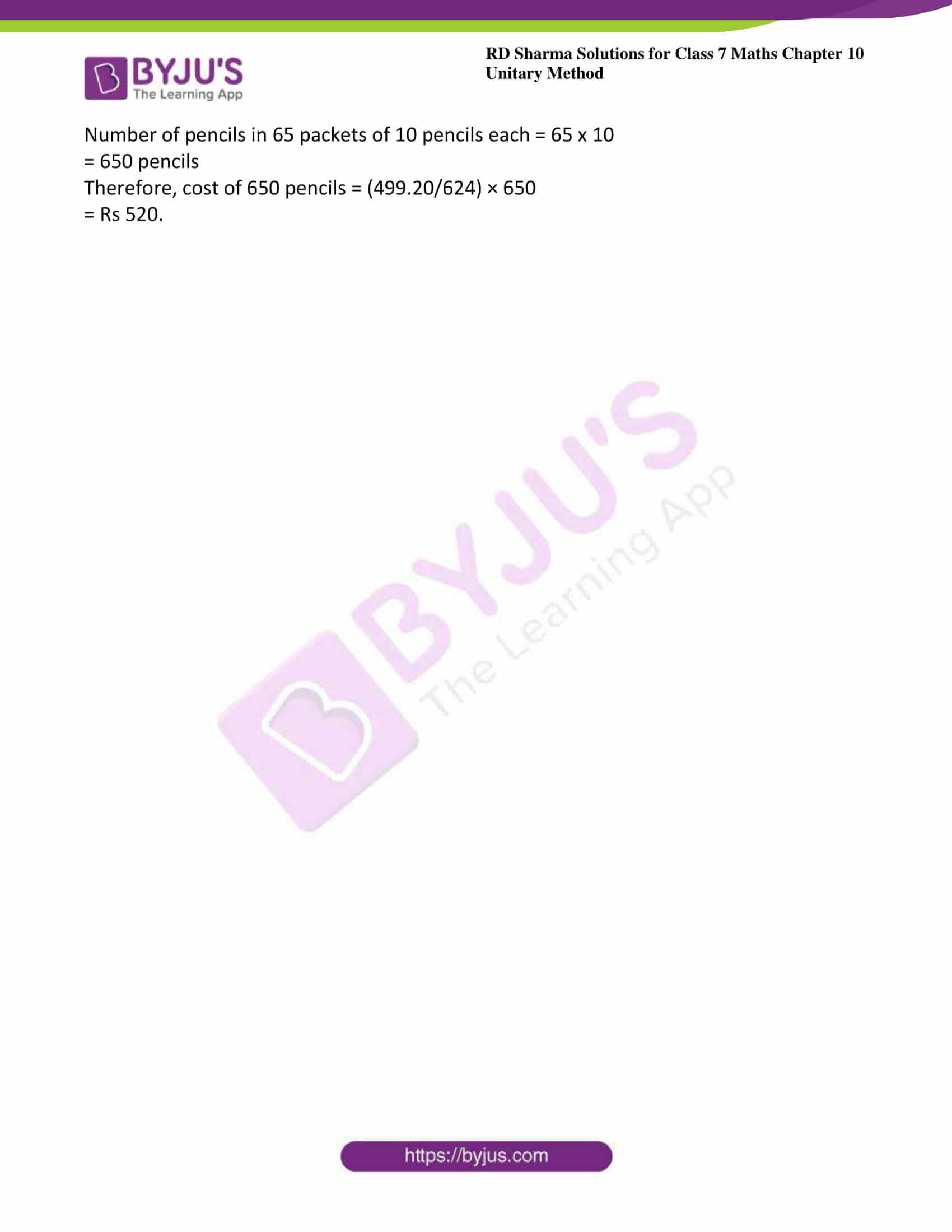# RD Sharma Solutions Class 7 Maths Chapter 10 Unitary Method Exercise 10.1

## Download the PDF of RD Sharma For Class 7 Maths Chapter 10 Unitary Method### Access answers to Maths RD Sharma Solutions For Class 7 Chapter 10 – Unitary Method

Exercise 10.1 Page No: 10.2

1. 20 chocolates cost Rs 320. Find the cost of 35 such chocolates.

Solution:

Given cost of 20 chocolates = Rs 320

Cost of 1 chocolate = (320/20)

Therefore, the cost of 35 chocolates = (320/20) × 35

= Rs 560

2. The cost of 40 meters of cloth is Rs 200. Find the cost of 50 meters of cloth.

Solution:

Given cost of 40 meters of cloth = Rs 200

Cost of 1 meter of cloth = (200/40)

Therefore, the cost of 50 chocolates = (200/40) × 50

= Rs 250

3. A car can cover a distance of 522 km on 36 litres of petrol. How far can it travel on 14 litres of petrol?

Solution:

Given that number of kilometres a car can cover by using 36 litres of petrol = 522 km

Number of kilometres a car can cover by using 1 litre of petrol = 522/36

Hence, the number of kilometres a car can cover by using 14 litres = (522/36) × 14

= 203 km

4. Travelling 900 km by rail costs Rs 280. What would be the fare for a journey of 360 km when a person travels by the same class?

Solution:

Given that cost of travelling 900 km by rail = Rs 280

Therefore cost of travelling 1 km by rail = (280/900)

Hence, Cost of travelling 360 km by rail = (280/900) × 360

= Rs 112

5. If 6 oil tankers can be filled by a pipe in 4(1/2) hours, how long does the pipe take to fill 4 such oil tankers?

Solution:

Given that time taken by 6 oil tankers to be filled by a pipe = 4 (1/2) hours = (9/2) hours

Time taken by 1 oil tanker to be filled by a pipe = (9/2)/6 hours = 9/ (2 x 6) = 9/12 hours

Hence time taken by 4 oil tankers to be filled by a pipe = (9/12) × 4

= 3 hours

6. 3/4 of the salary per month is Rs 600. What is the salary per month?

Solution:

Given that 3/4 of the salary per month = 600

Let the salary of the month be x

Therefore ¾ × x = 600

x = 600 × (4/3)

x = 800

Therefore salary per month is Rs 800

7. The cost of 32 tables is Rs 23520. Find the number of such tables that can be purchased for Rs 51450.

Solution:

Given that number of tables bought for Rs 23520 = 32

Number of tables bought for Rs 1 = 32/23520

Hence, number of tables bought for Rs 51450 = (32/23520) × 51450 = 70

8. The yield of wheat from 6 hectares is 280 quintals. Find the number of hectares required for a yield of 225 quintals.

Solution:

Given number of hectares required for a yield of 280 quintals = 6 hectares

Number of hectares required for a yield of 1 quintal = 6/280 hectares

Hence, the number of hectares required for a yield of 225 quintals = 6/280 × 225

= 4 23/28 hectares

9. Fifteen post cards cost Rs 2.25. What will be the cost of 36 post cards? How many postcards can we buy in Rs 45?

Solution:

Given cost of 15 post cards = Rs 2.25

Cost of 1 post card is = 2.25/15

Hence, the cost of 36 post cards = (2.25/15) × 36

= Rs 5.40

Number of post cards bought for Rs 2.25 = 15

Numbers of post cards bought for Rs 1 = 15/2.25

Hence number of post cards bought for Rs 45 = (15/2.25) × 45

= 300

10. A rail journey of 75 km costs Rs 215. How much will a journey of 120 km cost?

Solution:

Given cost of a rail journey of 75 km = Rs 215

Cost of a rail journey of 1 km = 215/75

Hence, cost of a rail journey of 120 km = (215/75) × 120

= Rs 344

11. If the sales tax on a purchase worth Rs 60 is Rs 4.20. What will be the sales tax on the purchase worth of Rs 150?

Solution:

Given sales tax on the purchase worth of Rs 60 = Rs 4.20

Sales tax on the purchase worth of Rs 1 = Rs 4.2060

Hence, sales tax on the purchase worth of Rs 150 = (4.20/60) × 150

= Rs 10.50

12. 52 packets of 12 pencils each, cost Rs 499.20. Find the cost of 65 packets of 10 pencils each.

Solution:

Given total number of pencils in 52 packets of 12 pencils each = 52 × 12

= 624 pencils

Also given that cost of 624 pencils = Rs 499.20

Cost of 1 pencil = (499.20/624)

Number of pencils in 65 packets of 10 pencils each = 65 x 10

= 650 pencils

Therefore, cost of 650 pencils = (499.20/624) × 650

= Rs 520.